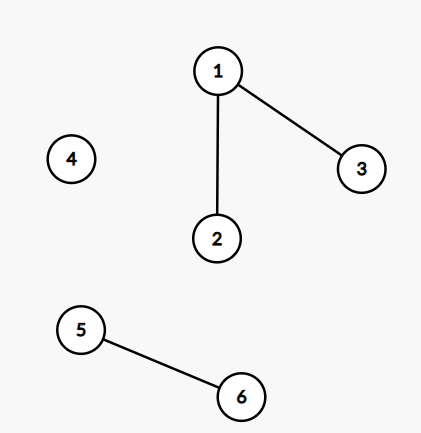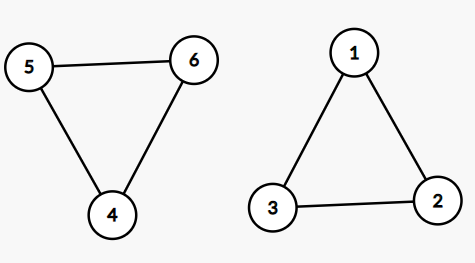# Minimum Cost to connect the graph by choosing any vertices that have a cost of at least 0

## Introduction

The problem states that we are given a graph, let say G, with N vertices and M edges. We are also given a cost[] for each vertex. The task is to connect this undirected graph in minimum cost, and the cost of connecting two vertices, let say, i and j, is the cost [i] + cost [j]. We can only join two vertices if the cost of vertices is at least 0, if it is not possible to connect two vertices, print -1; otherwise, print the minimum cost.

### Sample Examples

Example 1:-

Input:

N = 6.

Let the given graph be:Cost[]{2, 1, 5, 3, 2, 9}

Output

Explanation:

We connect Node 2 and Node 4, cost = 1 + 3 = 4.

And We will connect Node 2 and Node 5 = 1 + 2 = 3.

Now we have connect the graph , and total cost = 4 + 3 = 7.

Example 2:-

Input:

N = 6

Let the given graph be:Cost[] = {2, 1, 5, -3, -2, -9}

Output : -1

Explanation: It is impossible to connect the graph, as the cost for Node 4, Node 5, and Node 6 are less than 0.

## Solution Approach(Disjoint Set Union)

This problem will be solved using the Disjoint Set Union data Structure. The idea is to store all the minimum values greater than 0 of each connected component of a graph in a set. If any value among these minimum values is less than 0, then print -1, find the minimum value among all these minimum values, say minStore, and then find the sum of all the minimum values with this minStore and print the sum. Hence this will be minimum cost to connect the graph.

### Algorithm

1. Make parent, rank, and minVal array to store each node's parent, compare the ranks, and find the minimum element of each connected component, respectively.
2. Initialize the parent array as parent[i] = i, and minVal[i] = cost[i-1] in range [1,N].
3. Find the union of each edge of the graph by calling the function UnionTwoNode(x,y) for every pair of nodes.
4. Store the value of the parent array into unordered_set, let say s, so as to get the parent of each connected component.
5. Iterate over the unordered_set s, find the minimum value of s, store it in minStore and keep a check if any is negative.
6. If the graph is connected, print 0 if there is a negative value print -1.
7. Store the sum of each element from the unordered_set and minStore variable in the ans variable.
8. Finally, print the and variable.

### Implementation in C++

``````// C++ function to find the minimum cost to connect the graph
#include <bits/stdc++.h>
using namespace std;
// function to find the parent of the node in a graph
int findParent(int x, int *parent)
{
if (parent[x] == x)
return x;
return findParent(parent[x], parent);
}
// function to find the union of the two nodes
void UnionTwoNodes(int *parent, int *rank, int *minVal, int x, int y)
{
// Finding parent of Node x
x = findParent(x, parent);
// Finding parent of Node y
y = findParent(y, parent);
// If rank of both the nodes are same, simply increase rank of first node
if (rank[x] == rank[y])
rank[x]++;

if (rank[x] > rank[y])
{
parent[y] = x;
// updating the minVal array to store the minValue greater than 0.
if (minVal[x] < 0 && minVal[y] < 0)
{
minVal[x] = max(minVal[x], minVal[y]);
}
else if (minVal[x] < 0 && minVal[y] >= 0)
{
minVal[x] = minVal[y];
}
else if (minVal[x] >= 0 && minVal[y] >= 0)
{
minVal[x] = min(minVal[x], minVal[y]);
}
}
else
{
parent[x] = y;
// updating the minVal array to store the minValue greater than 0.
if (minVal[x] < 0 && minVal[y] < 0)
{
minVal[y] = max(minVal[x], minVal[y]);
}
else if (minVal[x] >= 0 && minVal[y] < 0)
{
minVal[y] = minVal[x];
}
else if (minVal[x] >= 0 && minVal[y] >= 0)
{
minVal[x] = min(minVal[x], minVal[y]);
}
}
}
// function to find the minimum cost to connect the graph
int findMinCost(vector<pair<int, int>> &graph, int *cost, int n, int m)
{
// declaring parent array to store the parent for every node,
// initially every node is its own parent
int *parent = new int[n + 1];
// rank array to store the rank of every node
int *rank = new int[n + 1];
// stores the minimum value of each set
int *minVal = new int[n + 1];
for (int i = 1; i <= n; i++)
{
// initially every node is its own parent
parent[i] = i;
// initially rank of every node is 0.
rank[i] = 0;
minVal[i] = cost[i - 1];
}
for (auto it = graph.begin(); it != graph.end(); it++)
{
// grouping the nodes that are connected, by making their parent Node same
UnionTwoNodes(parent, rank, minVal, it->first, it->second);
}

// map to store parent of each connected component
unordered_set<int> s;
for (int i = 1; i <= n; i++)
{
s.insert(parent[i]);
}

// variable to store the min value for the set with its index.
pair<int, int> minStore = {0, INT_MAX};
// flag variable that keep the check if minimum value is not less than 0.
// if less than 0, then true, otherwise false.
bool flag = false;
for (auto it = s.begin(); it != s.end(); it++)
{
// if minVal is less than 0,
if (minVal[*it] < 0)
{
// mark flag as true
flag = true;
}
if (minStore.second > minVal[*it])
{
minStore.first = *it;
minStore.second = minVal[*it];
}
}
// it will store the final answer, minimum cost to add the edges
int ans = 0;
if (flag == false)
{
for (auto it = s.begin(); it != s.end(); it++)
{
if (*it != minStore.first)
{
ans += (minVal[*it] + minStore.second);
}
}
}
else if (flag && s.size() == 1)
{
ans = 0;
}
else
{
ans = -1;
}
return ans;
}
int main()
{
int n = 6;
// initial given graph
vector<pair<int, int>> graph = {{1, 2}, {1, 3}, {5, 6}};
// cost of the vertex to be connected
int cost[] = {2, 5, 1, 3, 2, 9};
cout << findMinCost(graph, cost, n, graph.size()) << endl;
}``````

Output:

``7``

#### Complexity Analysis

Time Complexity: O(n*logM)

M is the number of edges and n is the number of nodes.

Space Complexity: O(n)

Since we have created only linear arrays of size n, so total space complexity is O(n) to find the minimum cost to connect the graph.

Q1. What is the difference between directed and undirected graph ?

Ans. The directed graph contains ordered pairs of vertices while the undirected contains unordered pairs of vertices. In a directed graph, edges represent the direction of vertices, while in unordered graph, edges do not represent the direction of vertices.

Q2. What is the maximum number of edges in the undirected graph of Nodes N?

Ans. Each node can have an edge with every other n-1 node in an undirected graph. Therefore the total number of edges possible are n*(n-1)/2.

Q3. Which data structure is used in the BFS and DFS of the graph?

Ans. In BFS, a queue data structure is used, while in DFS stack is used.

## Key takeaways

In this article, we have discussed the approach to connect the graph in minimum cost, by selecting vertex which have cost greater than 0. We hope you understand the problem and solution properly. Now you can do more similar questions.

If you are a beginner, interested in coding, and want to learn DSA, you can look for our guided path for DSA, which is free!j